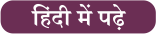Latest SSC jobs   »   Simple Interest Formula, Concept and Study...

# Simple Interest Formula, Concept and Study Notes

## Simple Interest

Simple Interest Formula: Simple Interest Formula, Concept, and Study Notes are provided below. Simple interest is the method by which you can calculate the interest on a loan. It is charged on the principal amount. In order to determine the simple interest, you need to multiply the principal amount with the rate of interest and the time period. It is calculated only on the original sum of money. Students must be aware of the simple interest formula to solve the questions asked in the exam. We are providing you with complete notes on simple interests.

## Simple Interest Formula

1. If a certain sum in T years at R% per annum amounts to Rs. A, then the sum will be

2. The annual payment that will discharge a debt of Rs. A due in T years at R% per annum is .

Annual payment=##### Click Here to Attempt More Questions From Quantitative Aptitude For SSC CGL

3. If a certain sum is invested in n types of investments in such a manner that equal amount is obtained on each investment where interest rates are R₁, R₂, R₃ ……, R_n, respectively and time periods are T₁, T₂, T₃, ……, T_n, respectively, then the ratio in which the amounts are invested is

4. If a certain sum of money becomes n times itself in T years at simple interest, then the rate of interest per annum is

5. If a certain sum of money becomes n times itself at R% per annum simple interest in T years, then

6. If a certain sum of money becomes n times itself in T years at simple interest, then the time T in which it will become m times itself is given by

7. Effect of change of P, R, and T on simple interest is given by the following formula:

8. If a certain sum of money P lent out at SI amounts to A₁ in T₁ years and to A₂ in T₂ years, then

9. If a certain sum of money P lent out for a certain time T amounts to A₁ at R₁ % per annum and to A₂ at R₂ % per annum, then

10. If an amount P₁ is lent at the simple interest rate of R₁ % per annum and another amount P₂ at the simple interest rate of R₂ % per annum, then the rate of interest for the whole sum is

## Simple Interest Formula: FAQ

Q. What is the use of Simple Interest?

Ans: Simple interest is used in cases where the amount to be returned requires a short period of time.

Q. What are the types of Simple Interests?

Ans: There are two types i.e. ordinary simple interest and exact simple interest.

Q. Are Home Loans Simple or Compound Interest?

Ans: A home loan based on the calculation of interest daily is called a simple-interest mortgage.

Q. Are Car Loans Simple or Compound Interest?

Ans: Interest on an auto loan is calculated using simple interest,

Q. What is the difference between Simple or Compound Interest?

Ans: Simple interest is based on the principal amount of a loan or deposit and compound interest is based on the principal amount and the interest that accumulates on it in every period.

Q. How do you calculate Simple Interest?

Ans: Simple Interest is calculated using the following formula: SI = P × R × T, where P = Principal, R = Rate of Interest, and T = Time period.

Q. How do I calculate Simple Interest monthly?

Ans: The formula for calculating monthly simple interest becomes (P × R × T) / (100 × 12).#### Congratulations!General Awareness & Science Capsule PDFIncorrect details? Fill the form again here

General Awareness & Science Capsule PDF

Thank You, Your details have been submitted we will get back to you.

### TOPICS:

•What is Frequency Polygon? Statistics, G...
•Speed, Time And Distance Tricks and Ques...
•Synonyms and Antonyms, List and Examples...
•Mensuration Formula And Questions for 2D...
•Pythagoras Theorem, Formula, Proof, Exam...
•Study Notes For Error Detection: Points ...